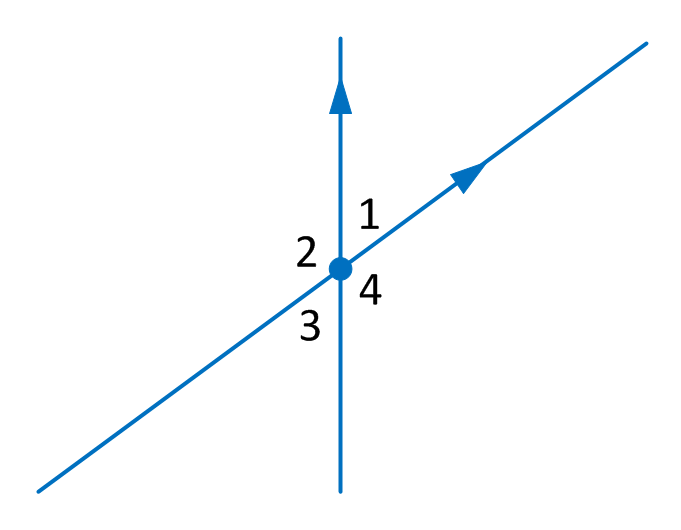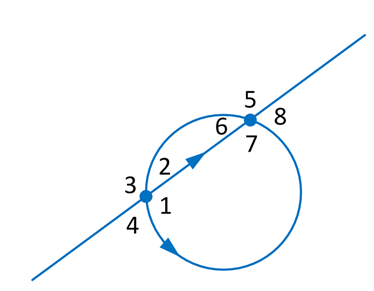# Objects and Functions¶

## Scalar:Distance¶

Scalar:Distance(<point>, <point>)

Distance between 2 Points:

```d = Scalar:Distance(P1, P2)
```
Distances from Point to Point are always positive values.

Scalar:Distance(<point>, <line>)

Abstand zwischen einem Punkt und einer Geraden:

```d = Scalar:Distance(P1, L1)
```
Ergebnis is positiv, wenn der Punkt in Fahrtrichtung rechts der Geraden liegt
Ergebnis ist negativ, wenn der Punkt in Fahrtrichtung links der Geraden liegt

Scalar:Distance(<point>; <circle>)

Abstand zwischen einem Punkt und einem Kreis:

```d = Scalar:Distance(P1, C1)
```
Ergebnis ist positiv, wenn der Punkt ausserhalb des Kreises liegt n
Ergebnis ist negativ, wenn der Punkt innerhalb des Kreises liegt

## Scalar:Angle¶

Scalar:Angle(<line>, <line>)

Angle (in degrees) between 2 lines:

```alfa = Scalar:Angle(L1, L2)
```
Ergebnis ist der erforderliche Winkel, um L1 fahrtrichtungsgleich auf L2 zu drehen.
(-180° <= Ergebnis < 180°).

## Point: Direct Creation¶

Point:Create(<number>, <number>)

Definition using x/y coordinates:

```P = Point:Create(x, y)
```
x: x coordinate
y: y coordinate

## Point: Intersections¶

### Intersection of lines¶

Point:Intersection(<line>, <line>)

Definition by intersection of 2 lines:

```P = Point:Intersection(L1, L2)
```
L1: first line object
L2: second line object

### Intersection of line and circle¶

Point:Intersection(<line>, <circle>, <number>)

Definition by intersection of a line and a circle:

```P = Point:Intersection(L1, C1, n)
```
L1: line object
C1: circle object
n: selected number of intersection: 1 (first), 2 (second)

### Intersection of 2 circles¶

Point:Intersection(<circle>, <circle>, <number>)

Definition by intersection of 2 circles:

```P = Point:Intersection(C1, C2, n)
```
C1: circle object
C2: circle object
n: selected number of intersection: 1 (first), 2 (second)

Reference line is the connection between the center points of the 2 circles.

## Point: Perpendiculars¶

### Perpendicular of a point to a line¶

Point:Perpendicular(<point>, <line>)

Definition by the intersection of perpendicular line from a point to the target line:

```Px = Point:Perpendicular(P1, L1)
```
P1: point object
L1: target line object

### Perpendicular of a point to a circle¶

Point:Perpendicular(<point>, <circle>)

Definition by the intersection of perpendicular line from a point to the target circle:

```Px = Point:Perpendicular(P1, C1)
```
P1: point object
C1: target circle object

## Point: Vanishing¶

Point:Align(<point>, <point>, <number>)

Definition by the vanishing point on the line between 2 points:

```P = Point:Align(P1, P2, rdist)
```
P1: first point object
P2: second point object
rdist: position of target point in relation to distance between P1 and P2
rdist < 0 : before P1
rdist == 0 : P1
0 < rdist < 1 : between P1 and P2
rdist == 1: P2
rdist > 1 : after P2

## Point: Reflections¶

Point:Mirror(<line> [,<line>]*, <point> [,<point]*)

Definition by the reflection point(s) on the line(s):

```Pa, Pb, Pc, Pd = Point:Mirror(L1, P1, P2, L2)
```
Pa: reflected point P1 on line L1
Pb: reflected point P2 on line L1
Pc: reflected point P1 on line L2
Pd: reflected point P2 on line L2

## Point:: Tangents¶

### Tangent from a point to a circle¶

Point:Tangent(<point>, <circle>, <number>)

Definition of a tangent point from a point to a circle:

```P = Point:Tangent(P1, C1, n)
```
P1: point object
C1: circle object
n: integer number 1 (right hand side), 2 (left hand side)

Reference direction is the line from P1 to the center of C1

### Tangent between 2 circles¶

Point:Tangent(<circle>, <circle>, <number>)

Definition of a tangent point of 2 circles:

```P = Point:Tangent(C1, C1, n)
```
C1: first circle object
C2: second circle object
n: integer number
1 right hand side on C1
2 right hand side on C2
3 left hand side on C1
4 left hand side on C2

Reference direction is the line from the center of C1 to the center of C2

## Point: Fillets (Rounding of corners)¶

### Rounding between 2 lines¶

Point:Rounding(<line>, <line>, <radius>, <number>)

Definition of a fillet between 2 lines:

```P1, P2, P3 = Point:Rounding(L1, L2, r, n)
```P1: center point of arc circle P2: starting point of arc circle P3: ending point of arc circle r: radius of arc circle n: selection of area as shown in figure (returns all results if < 1)

### Rounding between line and circle¶

Point:Rounding(<line>, <circle>, <radius>, <number>)

Definition of a fillet between a line and a circle:

```P1, P2, P3 = Point:Rounding(L1, C1, r, n)
```P1: center point of arc circle P2: starting point of arc circle P3: ending point of arc circle r: radius of arc circle n: selection of area as shown in figure (returns all results if < 1)

### Rounding between 2 circles¶

Point:Rounding(<circle>, <circle>, <radius>, <number>)

Definition of a fillet between 2 circles:

```P1, P2, P3 = Point:Rounding(C1, C2, r, n)
```P1: center point of arc circle P2: starting point of arc circle P3: ending point of arc circle r: radius of arc circle n: selection of area as shown in figure (returns all results if < 1)

## Line: Create¶

### 2 Points¶

Line:Create(<point>, <point>)

Definition by 2 points:

```L = Line:Create(P1, P2)
```
P1: first point
P2: second point

### Point and Angle¶

Line:Create(<point>, <angle>)

Definition by one point and an angle:

```L = Line:Create(P1, alfa)
```
P1: first point
angle: angle from x-axis in degree

### Point and Perpendicular¶

Line:Create(<point>, <line>)

Definition by a point and a perpendicular line:

```L = Line:Create(P1, L1)
```
P1: point which must not be part of the target line
L1: perpendicular line

### Perpendicular of line between 2 Points¶

Line:Perpendicular(<point>, <point>, <rdist>)

Definition by a point and a perpendicular line:

```L = Line:Perpendicular(P1, L1, rdist)
```
P1: first point
P2: second point
rdist: position of target line rotated by 90 deg in relation to distance between P1 and P2
rdist < 0 : before P1
rdist == 0 : P1
0 < rdist < 1 : between P1 and P2
rdist == 1: P2
rdist > 1 : after P2

### Parallel line of point¶

Line:Parallel(<line>, <point>)

Definition by a parallel line and a point:

```L = Line:Parallel(L1, P1)
```
L1: line
P1: point of the target parallel line

### Parallel line with distance¶

Line:Parallel(<line>, <distance>)

Definition by a parallel line and a distance:

```L = Line:Parallel(L1, dist)
```
L1: line
dist: distance of the target parallel line (>0 right hand side, <0 left hand side)

## Circle¶

### Direct construction¶

Definition by center point and radius:

```C = Circle:Create(P1, rad)
```
P1: center point

### Center and Radius¶

Definition by center point and radius:

```C = Circle:Create(P1, rad)
```
P1: center point
```C = Circle:Create(P1, P2, P3)